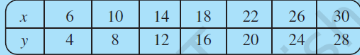# Q1. Observe the given table and find if x and y are directly proportional.If x and y are directly proportional then   must be equal to a constant value let say some  'k'.

Hence we then calculating:

,

as you can see that all these values are not equal hence,

we can say that x and y are not directly proportional.

Exams
Articles
Questions